The HPSUMMARY Procedure

Computational Resources

The total of unique classification values that PROC HPSUMMARY allows depends on the amount of computer memory that is available. PROC HPSUMMARY uses the same memory allocation scheme across all operating environments. When classification variables are involved, PROC HPSUMMARY must keep a copy of each unique value of each classification variable in memory. You can estimate the memory requirements to group the classification variable by calculating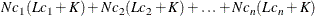where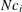is the number of unique values for the classification variable,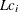is the combined unformatted and formatted length of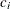, and K is some constant on the order of 32 bytes (64 for 64-bit architectures). When you use the GROUPINTERNAL option in the CLASS statement,is simply the unformatted length of.

The GROUPINTERNAL option can improve computer performance because the grouping process is based on the internal values of the classification variables. If a numeric classification variable is not assigned a format and you do not specify GROUPINTERNAL, then PROC HPSUMMARY uses the default format, BEST12., to format numeric values as character strings. Then PROC HPSUMMARY groups these numeric variables by their character values, which takes additional time and computer memory.

Each unique combination of classification variables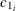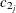for a given type forms a level in that type. See the section TYPES Statement. You can estimate the maximum potential space requirements for all levels of a given type, when all combinations actually exist in the data (a complete type), by calculating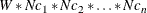where W is a constant based on the number of variables analyzed and the number of statistics calculated (unless you request QMETHOD= OS to compute the quantiles) and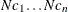are the number of unique levels for the active classification variables of the given type.

Clearly, the memory requirements of the levels overwhelm the levels of the classification variables. For information about how to adjust your computation resource parameters, see the SAS documentation for your operating environment.

Another way to enhance performance is by carefully applying the TYPES or WAYS statement, limiting the computations to only those combinations of classification variables that you are interested in.